Home » Why Flow Directly Proportional to Square Root of ΔP ?
Flow Measurement

# Why Flow Directly Proportional to Square Root of ΔP ?

Flow element used to create a pressure change by accelerating a fluid stream is the venturi tube (Here Differential Pressure based flow meter i.e.Venturi tube is taken as an example for discussion): a pipe purposefully narrowed to create a region of low pressure.

As discussed previously, venturi tubes are not the only structure capable of producing a flow-dependent pressure drop. You should keep this in mind as we proceed to derive equations relating flow rate with pressure change: although the venturi tube is the canonical form, the exact same mathematical relationship applies to all flow elements generating a pressure drop by accelerating fluid, including orifice plates, flow nozzles, V-cones, segmental wedges, pipe elbows, pitot tubes, etc.

If the fluid going through the venturi tube is a liquid under relatively low pressure, we may vividly show the pressure at different points in the tube by means of piezometers (Think of a piezometer tube as nothing more than a manometer tube: the greater the fluid pressure at the bottom of the tube, the higher the liquid will rise inside the tube.), which are transparent tubes allowing us to view liquid column heights.

The greater the height of liquid column in the piezometer, the greater the pressure at that point in the flowstream: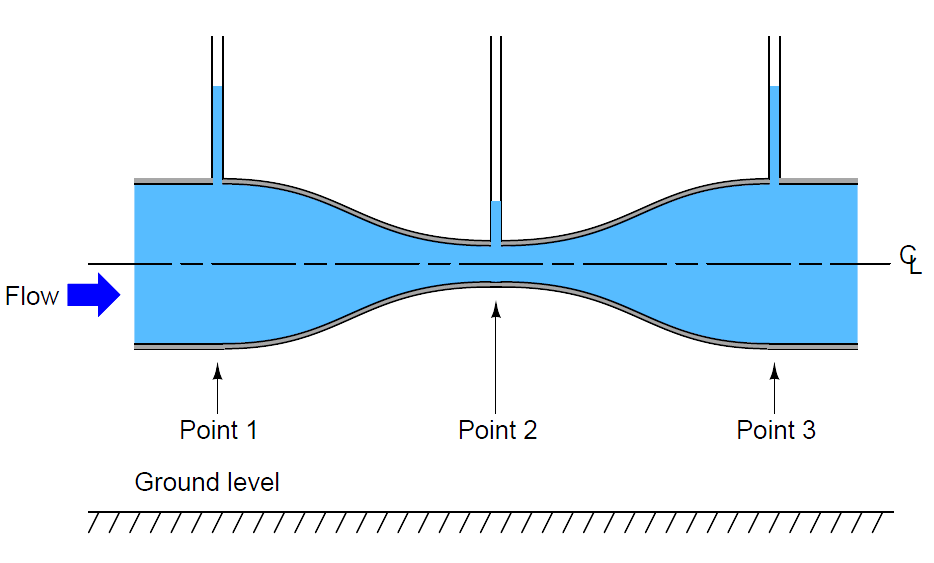As indicated by the piezometer liquid heights, pressure at the constriction (point 2) is the least, while pressures at the wide portions of the venturi tube (points 1 and 3) are the greatest.

This is a counter-intuitive result, but it has a firm grounding in the physics of mass and energy conservation. If we assume no energy is added (by a pump) or lost (due to friction) as fluid travels through this pipe, then the Law of Energy Conservation describes a situation where the fluid’s energy must remain constant at all points in the pipe as it travels through. If we assume no fluid joins this flow stream from another pipe, or is lost from this pipe through any leaks, then the Law of Mass Conservation describes a situation where the fluid’s mass flow rate must remain constant at all points in the pipe as it travels through.

So long as fluid density remains fairly constant, fluid velocity must increase as the cross-sectional area of the pipe decreases, as described by the Law of Continuity: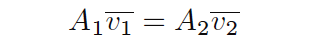Rearranging variables in this equation to place velocities in terms of areas, we get the following result: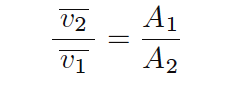This equation tells us that the ratio of fluid velocity between the narrow throat (point 2) and the wide mouth (point 1) of the pipe is the same ratio as the mouth’s area to the throat’s area.

So, if the mouth of the pipe had an area 5 times as great as the area of the throat, then we would expect the fluid velocity in the throat to be 5 times as great as the velocity at the mouth.

Simply put, the narrow throat causes the fluid to accelerate from a lower velocity to a higher velocity.

We know from our study of energy in physics that kinetic energy is proportional to the square of a mass’s velocity (Ek = (1/2)mv2). If we know the fluid molecules increase velocity as they travel through the venturi tube’s throat, we may safely conclude that those molecules’ kinetic energies must increase as well.

However, we also know that the total energy at any point in the fluid stream must remain constant, because no energy is added to or taken away from the stream in this simple fluid system.

Therefore, if kinetic energy increases at the throat, potential energy must correspondingly decrease to keep the total amount of energy constant at any point in the fluid.

Potential energy may be manifest as height above ground, and/or as pressure in a fluid system. Since this venturi tube is level with the ground, there cannot be a height change to account for a change in potential energy.

Therefore, there must be a change of pressure (P) as the fluid travels through the venturi throat. The Laws of Mass and Energy Conservation invariably lead us to this conclusion: fluid pressure must decrease as it travels through the narrow throat of the venturi tube.

Conservation of energy at different points in a fluid stream is neatly expressed in Bernoulli’s Equation as a constant sum of elevation, pressure, and velocity “heads”:Where,

z = Height of fluid (from a common reference point, usually ground level)
ρ = Mass density of fluid
g = Acceleration of gravity
v = Velocity of fluid
P = Pressure of fluid

We will use Bernoulli’s equation to develop a precise mathematical relationship between pressure and flow rate in a venturi tube. To simplify our task, we will hold to the following assumptions for our venturi tube system:

• No energy lost or gained in the venturi tube (all energy is conserved)
• No mass lost or gained in the venturi tube (all mass is conserved)
• Fluid is incompressible
• Venturi tube centerline is level (no height changes to consider)

Applying the last two assumptions to Bernoulli’s equation, we see that the “elevation head” term drops out of both sides, since z, ρ, and g are equal at all points in the system: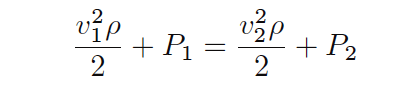Now we will algebraically re-arrange this equation to show pressures at points 1 and 2 in terms of velocities at points 1 and 2: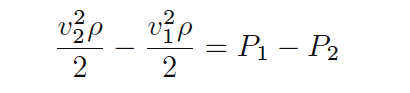Factoring ρ/2 out of the velocity head terms:The Continuity equation shows us the relationship between velocities v1 and v2 and the areas at those points in the venturi tube, assuming constant density (ρ):

#### A1v1 = A2v2

Specifically, we need to re-arrange this equation to define v1 in terms of v2 so we may substitute into Bernoulli’s equation:Performing the algebraic substitution: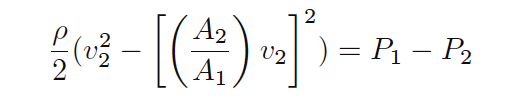Distributing the “square” power:Factoring v22 out of the outer parentheses set: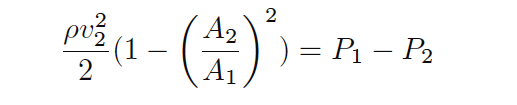Solving for v2, step by step: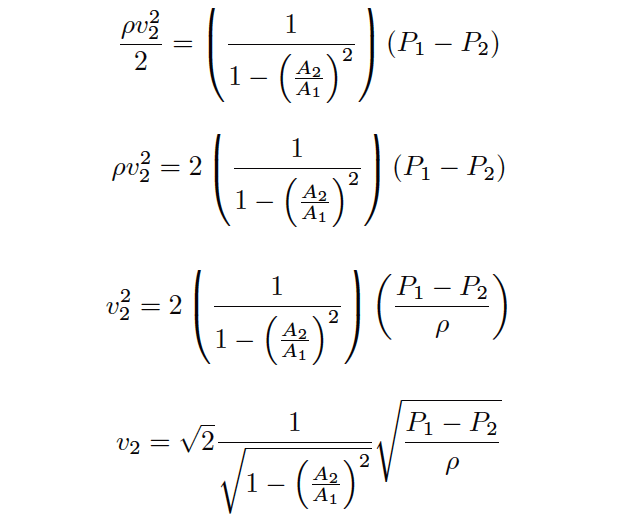The result shows us how to solve for fluid velocity at the venturi throat (v2) based on a difference of pressure measured between the mouth and the throat (P1 −P2).

We are only one step away from a volumetric flow equation here, and that is to convert velocity (v) into flow rate (Q).

Velocity is expressed in units of length per time (feet or meters per second or minute), while volumetric flow is expressed in units of volume per time (cubic feet or cubic meters per second or minute).

Simply multiplying throat velocity (v2) by throat area (A2) will give us the result we seek:

General flow/area/velocity relationship:

#### Q = Av

Equation for throat velocity: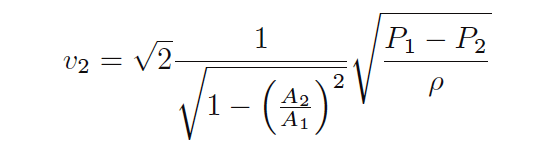Multiplying both sides of the equation by throat area: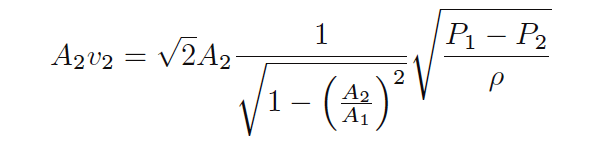Now we have an equation solving for volumetric flow (Q) in terms of pressures and areas: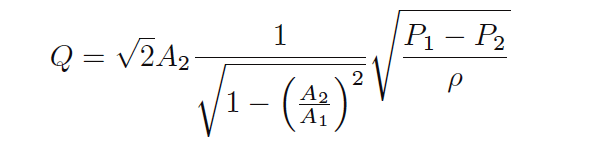Please note how many constants we have in this equation. For any given venturi tube, the mouth and throat areas (A1 and A2) will be fixed.

This means nearly half the variables found within this rather long equation are actually constant for any given venturi tube, and therefore do not change with pressure, density, or flow rate.

Knowing this, we may re-write the equation as a simple proportionality:To make this a more precise mathematical statement, we may insert a constant of proportionality (k) and once more have a true equation to work with: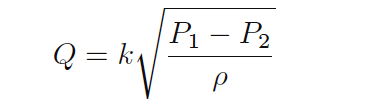There is a quadratic (“square”) relationship between velocity and differential pressure precisely because there is a quadratic relationship between velocity and kinetic energy as all first-quarter physics students learn (Ek = 1/2mv2).

This is why ΔP increases with the square of flow rate (Q2) and why we must “square-root” the ΔP signal to obtain a flow measurement.

This is also why fluid density is so important in the orifice-plate flow equation.

The denser a fluid is, the more work will be required to accelerate it through a constriction, resulting in greater ΔP, all other conditions being equal: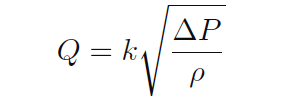The density (ρ) is  assumed as unity. Read the “Facts About Orifice Flow Meters” article for easy understanding the facts.

so the final equation will be :Generally we use the above simplified equation for all the differential pressure based flow meters like orifice, venturi, flow nozzles, V-cones, segmental wedges, pipe elbows, pitot tubes, etc. which is Flow Directly Proportional to Square Root of ΔP.

#### Articles You May Like :

Coriolis Flow Meter Inaccuracy

Turbine Flow Meter Installation

Thermal Dispersion Flow Switch

How to Select a Rotameter ?

Ultrasonic Flow meters Animation

#### What is Coriolis Flow Meter ?

This website uses cookies to improve your experience. We'll assume you're ok with this, but you can opt-out if you wish. Accept Read More

WordPress Image Lightbox

Send this to a friend